Скачать презентацию ELEC 2041 Microprocessors and Interfacing Lectures 22 Fractions

0b3ab1b4d34d10fb9a80383d68b5cb89.ppt

• Количество слайдов: 28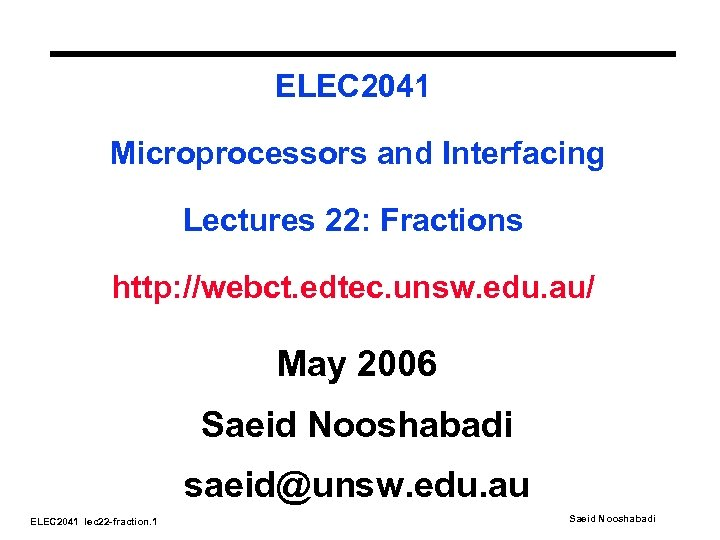ELEC 2041 Microprocessors and Interfacing Lectures 22: Fractions http: //webct. edtec. unsw. edu. au/ May 2006 Saeid Nooshabadi [email protected] edu. au ELEC 2041 lec 22 -fraction. 1 Saeid Nooshabadi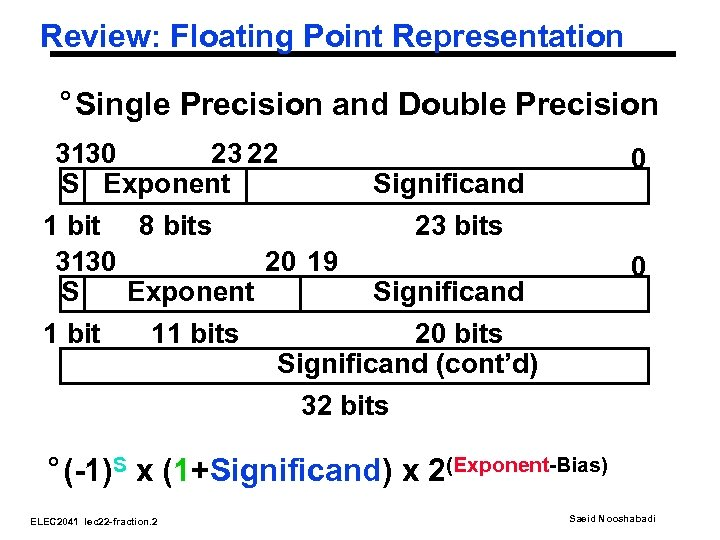Review: Floating Point Representation ° Single Precision and Double Precision 3130 23 22 S Exponent 1 bit 8 bits 3130 20 19 S Exponent 1 bit 11 bits 0 Significand 23 bits 0 Significand 20 bits Significand (cont’d) 32 bits ° (-1)S x (1+Significand) x 2(Exponent-Bias) ELEC 2041 lec 22 -fraction. 2 Saeid Nooshabadi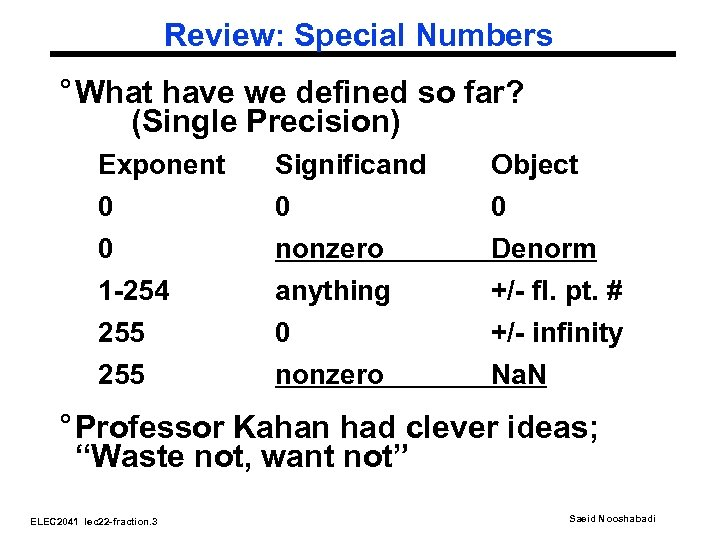Review: Special Numbers ° What have we defined so far? (Single Precision) Exponent Significand Object 0 0 nonzero Denorm 1 -254 anything +/- fl. pt. # 255 0 nonzero +/- infinity Na. N ° Professor Kahan had clever ideas; “Waste not, want not” ELEC 2041 lec 22 -fraction. 3 Saeid Nooshabadi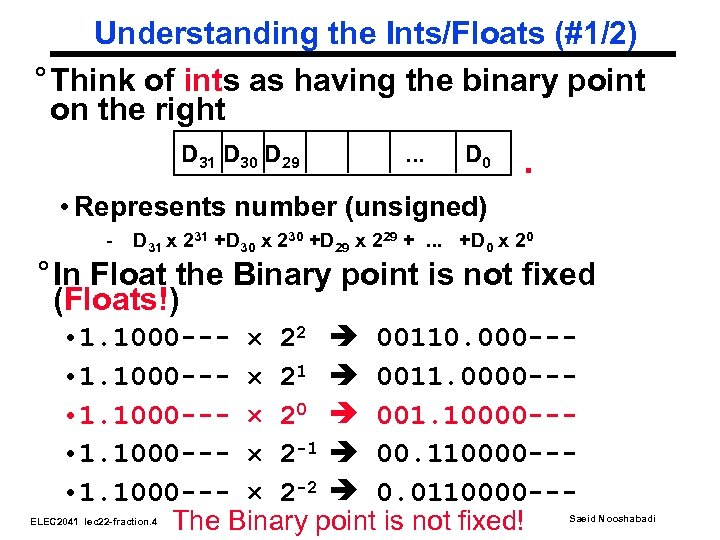Understanding the Ints/Floats (#1/2) ° Think of ints as having the binary point on the right D 31 D 30 D 29 . . . D 0 . • Represents number (unsigned) - D 31 x 231 +D 30 x 230 +D 29 x 229 +. . . +D 0 x 20 ° In Float the Binary point is not fixed (Floats!) • 1. 1000 --- 22 00110. 000 -- • 1. 1000 --- 21 0011. 0000 -- • 1. 1000 --- 20 001. 10000 -- • 1. 1000 --- 2 -1 00. 110000 -- • 1. 1000 --- 2 -2 0. 0110000 --The Binary point is not fixed! ELEC 2041 lec 22 -fraction. 4 Saeid Nooshabadi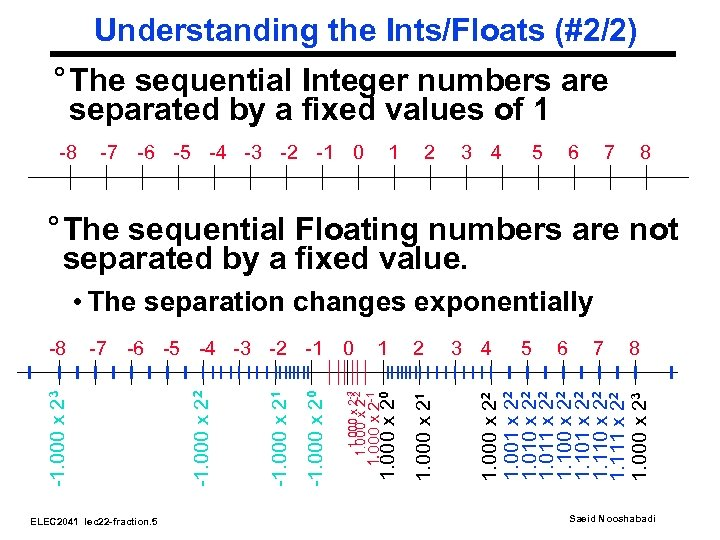Understanding the Ints/Floats (#2/2) ° The sequential Integer numbers are separated by a fixed values of 1 -8 -7 -6 -5 -4 -3 -2 -1 0 1 2 3 4 5 6 7 8 ° The sequential Floating numbers are not separated by a fixed value. ELEC 2041 lec 22 -fraction. 5 1. 000 x 21 1. 000 x 2 -3 2 3 4 5 6 7 8 1. 000 x 22 1. 001 x 22 1. 010 x 22 1. 011 x 22 1. 100 x 22 1. 101 x 22 1. 110 x 22 1. 111 x 22 1. 000 x 23 1 1. 000 x 2 -2 -1. 000 x 20 -1. 000 x 21 -1. 000 x 22 -7 -6 -5 -4 -3 -2 -1 0 -1. 000 x 23 -8 1. 000 x 20 • The separation changes exponentially Saeid Nooshabadi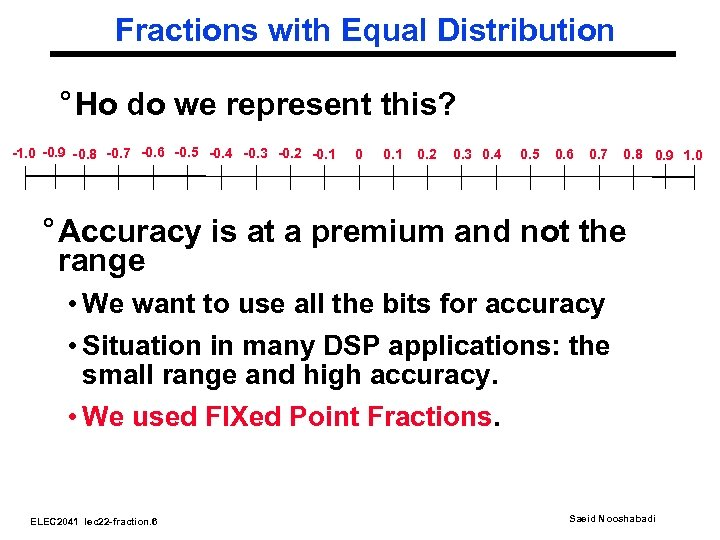Fractions with Equal Distribution ° Ho do we represent this? -1. 0 -0. 9 -0. 8 -0. 7 -0. 6 -0. 5 -0. 4 -0. 3 -0. 2 -0. 1 0. 2 0. 3 0. 4 0. 5 0. 6 0. 7 0. 8 0. 9 1. 0 ° Accuracy is at a premium and not the range • We want to use all the bits for accuracy • Situation in many DSP applications: the small range and high accuracy. • We used FIXed Point Fractions. ELEC 2041 lec 22 -fraction. 6 Saeid Nooshabadi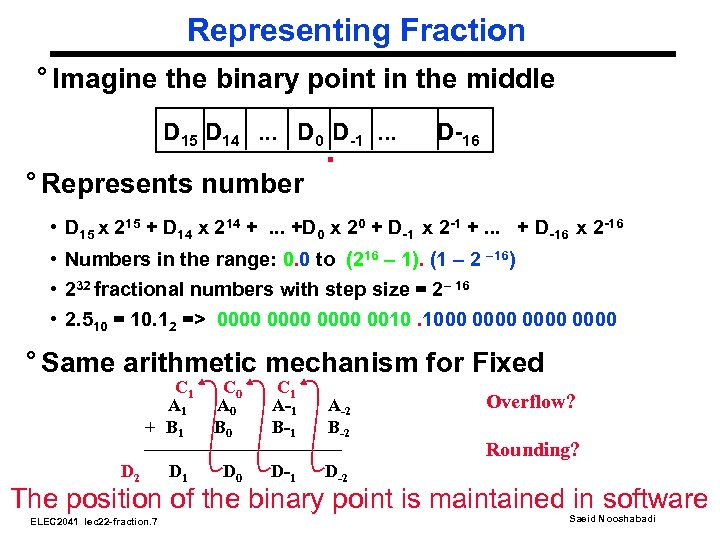Representing Fraction ° Imagine the binary point in the middle D 15 D 14. . . D 0 D-1. . . ° Represents number . D-16 • D 15 x 215 + D 14 x 214 +. . . +D 0 x 20 + D-1 x 2 -1 +. . . + D-16 x 2 -16 • Numbers in the range: 0. 0 to (216 – 1). (1 – 2 – 16) • 232 fractional numbers with step size = 2– 16 • 2. 510 = 10. 12 => 0000 0010. 1000 0000 ° Same arithmetic mechanism for Fixed C 1 A 1 + B 1 D 2 D 1 C 0 A 0 B 0 C 1 A-1 B-1 A-2 B-2 D 0 D-1 D-2 Overflow? Rounding? The position of the binary point is maintained in software ELEC 2041 lec 22 -fraction. 7 Saeid Nooshabadi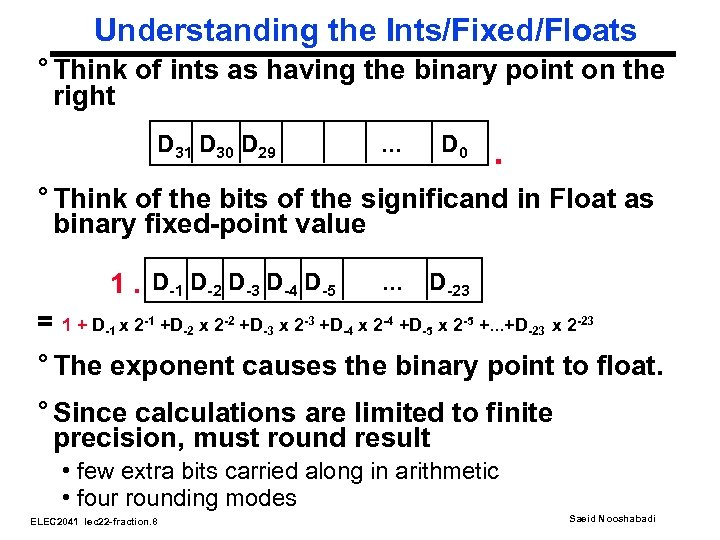Understanding the Ints/Fixed/Floats ° Think of ints as having the binary point on the right D 31 D 30 D 29 . . . D 0 . ° Think of the bits of the significand in Float as binary fixed-point value 1. D-1 D-2 D-3 D-4 D-5 . . . D-23 = 1 + D-1 x 2 -1 +D-2 x 2 -2 +D-3 x 2 -3 +D-4 x 2 -4 +D-5 x 2 -5 +. . . +D-23 x 2 -23 ° The exponent causes the binary point to float. ° Since calculations are limited to finite precision, must round result • few extra bits carried along in arithmetic • four rounding modes ELEC 2041 lec 22 -fraction. 8 Saeid Nooshabadi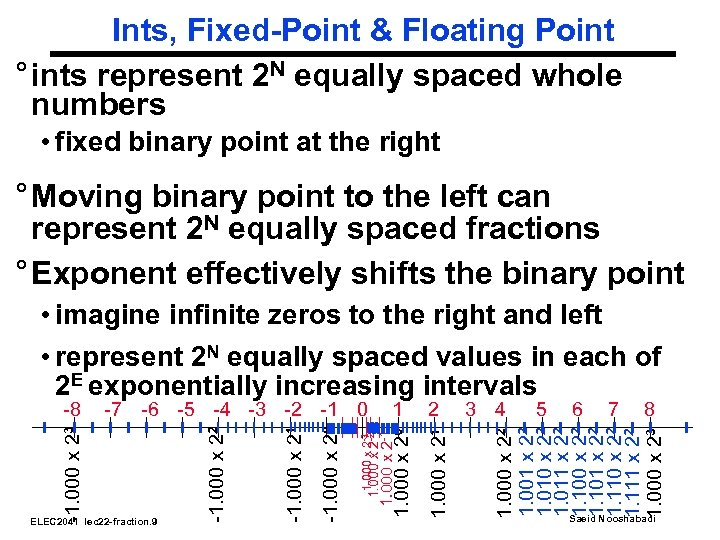Ints, Fixed-Point & Floating Point ° ints represent 2 N equally spaced whole numbers • fixed binary point at the right ° Moving binary point to the left can represent 2 N equally spaced fractions ° Exponent effectively shifts the binary point 3 4 5 6 7 8 1. 000 x 22 1. 001 x 22 1. 010 x 22 1. 011 x 22 1. 100 x 22 1. 101 x 22 1. 110 x 22 1. 111 x 22 1. 000 x 23 1. 000 x 21 1. 000 x 2 -3 2 1. 000 x 2 -1 1 1. 000 x 2 -2 -1. 000 x 20 ELEC 2041 lec 22 -fraction. 9 -1. 000 x 21 -7 -6 -5 -4 -3 -2 -1 0 -1. 000 x 22 -1. 000 x 23 -8 1. 000 x 20 • imagine infinite zeros to the right and left • represent 2 N equally spaced values in each of 2 E exponentially increasing intervals Saeid Nooshabadi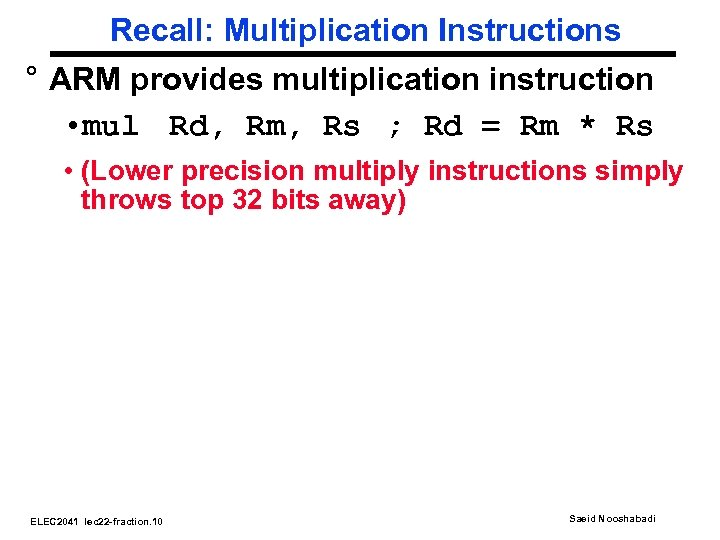Recall: Multiplication Instructions ° ARM provides multiplication instruction • mul Rd, Rm, Rs ; Rd = Rm * Rs • (Lower precision multiply instructions simply throws top 32 bits away) ELEC 2041 lec 22 -fraction. 10 Saeid Nooshabadi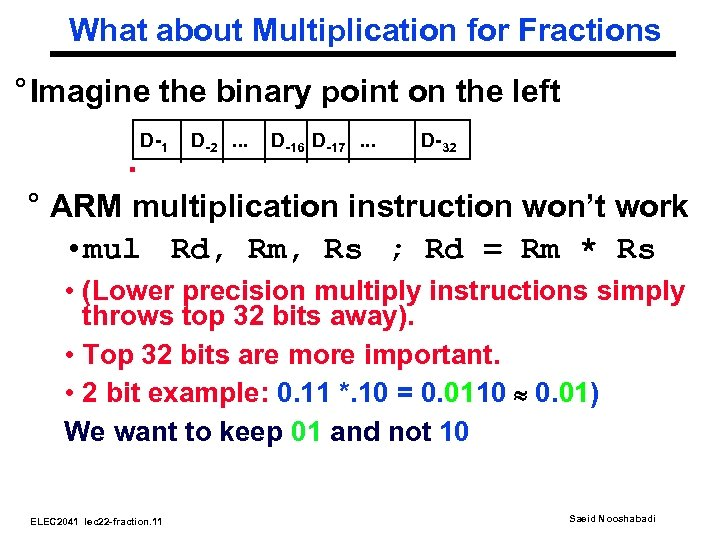What about Multiplication for Fractions ° Imagine the binary point on the left . D-1 D-2. . . D-16 D-17. . . D-32 ° ARM multiplication instruction won’t work • mul Rd, Rm, Rs ; Rd = Rm * Rs • (Lower precision multiply instructions simply throws top 32 bits away). • Top 32 bits are more important. • 2 bit example: 0. 11 *. 10 = 0. 0110 0. 01) We want to keep 01 and not 10 ELEC 2041 lec 22 -fraction. 11 Saeid Nooshabadi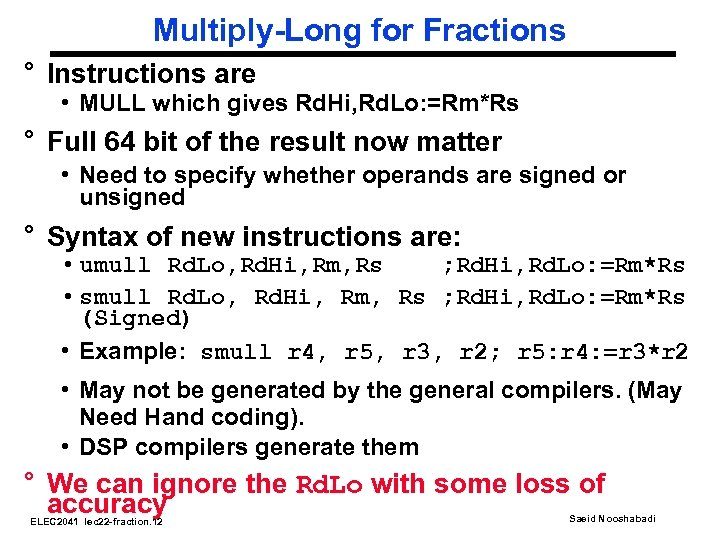Multiply-Long for Fractions ° Instructions are • MULL which gives Rd. Hi, Rd. Lo: =Rm*Rs ° Full 64 bit of the result now matter • Need to specify whether operands are signed or unsigned ° Syntax of new instructions are: • umull Rd. Lo, Rd. Hi, Rm, Rs ; Rd. Hi, Rd. Lo: =Rm*Rs • smull Rd. Lo, Rd. Hi, Rm, Rs ; Rd. Hi, Rd. Lo: =Rm*Rs (Signed) • Example: smull r 4, r 5, r 3, r 2; r 5: r 4: =r 3*r 2 • May not be generated by the general compilers. (May Need Hand coding). • DSP compilers generate them ° We can ignore the Rd. Lo with some loss of accuracy ELEC 2041 lec 22 -fraction. 12 Saeid Nooshabadi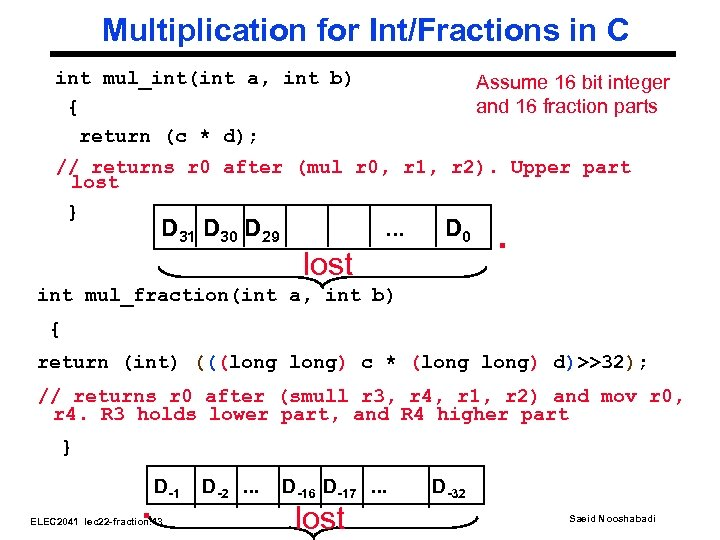Multiplication for Int/Fractions in C int mul_int(int a, int b) { return (c * d); Assume 16 bit integer and 16 fraction parts // returns r 0 after (mul r 0, r 1, r 2). Upper part lost } D 31 D 30 D 29 . . . D 0 lost . int mul_fraction(int a, int b) { return (int) (((long) c * (long) d)>>32); // returns r 0 after (smull r 3, r 4, r 1, r 2) and mov r 0, r 4. R 3 holds lower part, and R 4 higher part } . D-1 ELEC 2041 lec 22 -fraction. 13 D-2. . . D-16 D-17. . . lost D-32 Saeid Nooshabadi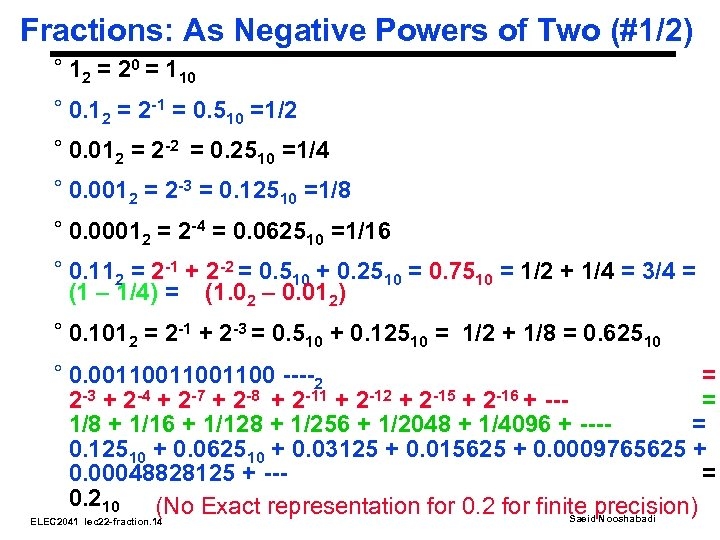Fractions: As Negative Powers of Two (#1/2) ° 12 = 20 = 110 ° 0. 12 = 2 -1 = 0. 510 =1/2 ° 0. 012 = 2 -2 = 0. 2510 =1/4 ° 0. 0012 = 2 -3 = 0. 12510 =1/8 ° 0. 00012 = 2 -4 = 0. 062510 =1/16 ° 0. 112 = 2 -1 + 2 -2 = 0. 510 + 0. 2510 = 0. 7510 = 1/2 + 1/4 = 3/4 = (1 – 1/4) = (1. 02 – 0. 012) ° 0. 1012 = 2 -1 + 2 -3 = 0. 510 + 0. 12510 = 1/2 + 1/8 = 0. 62510 ° 0. 0011001100 ----2 = 2 -3 + 2 -4 + 2 -7 + 2 -8 + 2 -11 + 2 -12 + 2 -15 + 2 -16 + --= 1/8 + 1/16 + 1/128 + 1/256 + 1/2048 + 1/4096 + ---= 0. 12510 + 0. 062510 + 0. 03125 + 0. 015625 + 0. 0009765625 + 0. 00048828125 + --= 0. 210 (No Exact representation for 0. 2 for finite precision) Saeid Nooshabadi ELEC 2041 lec 22 -fraction. 14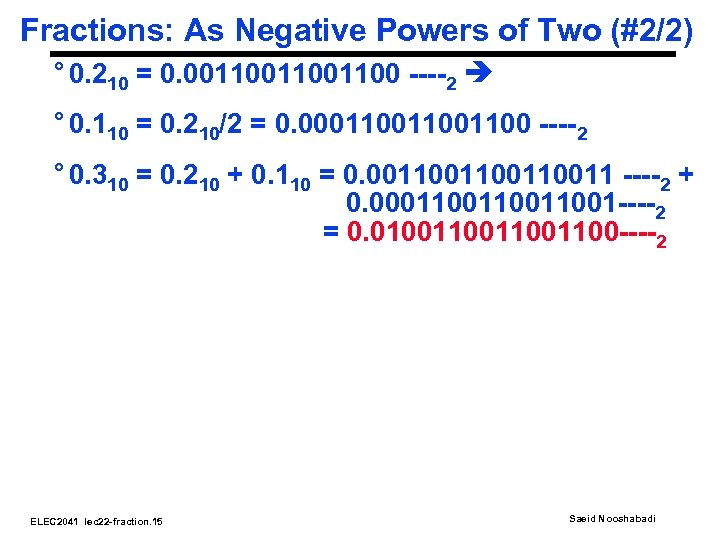Fractions: As Negative Powers of Two (#2/2) ° 0. 210 = 0. 0011001100 ----2 ° 0. 110 = 0. 210/2 = 0. 00011001100 ----2 ° 0. 310 = 0. 210 + 0. 110 = 0. 00110011 ----2 + 0. 000110011001 ----2 = 0. 010011001100 ----2 ELEC 2041 lec 22 -fraction. 15 Saeid NooshabadiToys & Tools YOU NEVER KNEW YOU NEEDED IT TVs that light up the room—even when they’re off ° Philips Electronics NV this month began shipping flat-screen televisions that are also room lights. Philips’s Ambilight technology projects background light from the rear of the television onto the wall, creating a halo around the television, which softly lights the room. The viewer can adjust the color choice and brightness via remote control (whether the television itself is on or off). Or the system can be set to an automatic mode, in which the lighting is continuously adjusted in relation to the image on the screen and to the overall brightness of the room, determined by built-in sensors. Available in 50 -inch plasma Flat. TV \$US 10 000 ELEC 2041 lec 22 -fraction. 16 Saeid Nooshabadi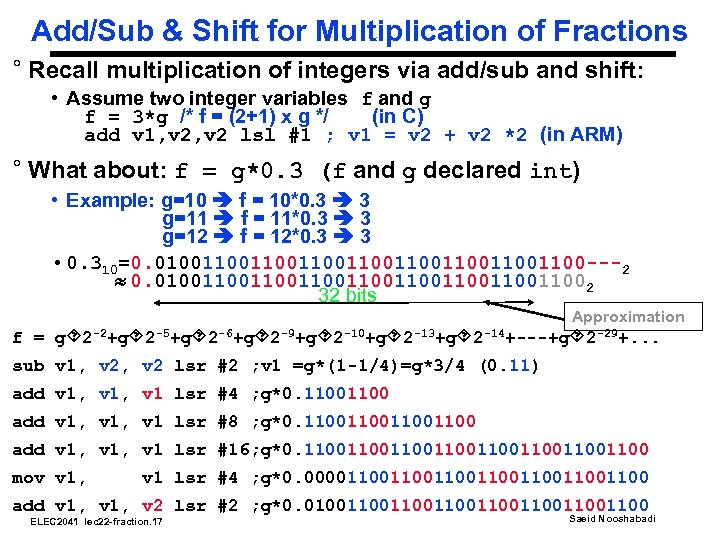Add/Sub & Shift for Multiplication of Fractions ° Recall multiplication of integers via add/sub and shift: • Assume two integer variables f and g f = 3*g /* f = (2+1) x g */ (in C) add v 1, v 2 lsl #1 ; v 1 = v 2 + v 2 *2 (in ARM) ° What about: f = g*0. 3 (f and g declared int) • Example: g=10 f = 10*0. 3 3 g=11 f = 11*0. 3 3 g=12 f = 12*0. 3 3 • 0. 310=0. 01001100110011001100 ---2 0. 010011001100110011002 32 bits Approximation f = g 2 -2+g 2 -5+g 2 -6+g 2 -9+g 2 -10+g 2 -13+g 2 -14+---+g 2 -29+. . . sub v 1, v 2 lsr #2 ; v 1 =g*(1 -1/4)=g*3/4 (0. 11) add v 1, v 1 lsr #4 ; g*0. 1100 add v 1, v 1 lsr #8 ; g*0. 11001100 add v 1, v 1 lsr #16; g*0. 1100110011001100 mov v 1, v 1 lsr #4 ; g*0. 00001100110011001100 add v 1, v 2 lsr #2 ; g*0. 01001100110011001100 ELEC 2041 lec 22 -fraction. 17 Saeid Nooshabadi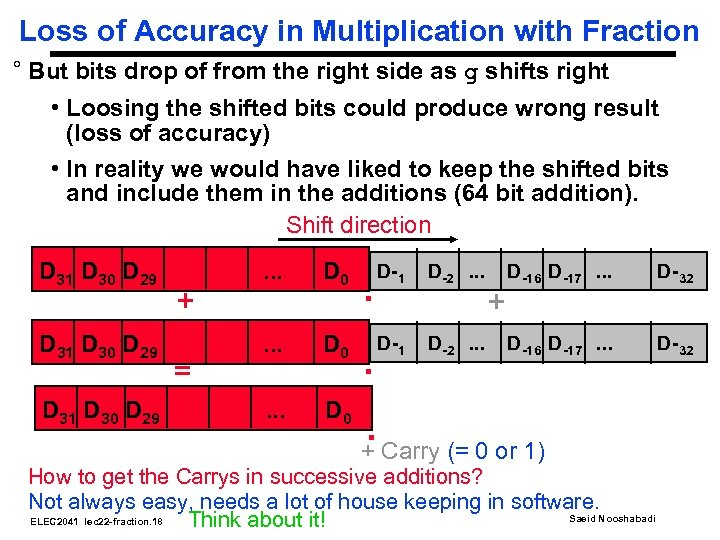Loss of Accuracy in Multiplication with Fraction ° But bits drop of from the right side as g shifts right • Loosing the shifted bits could produce wrong result (loss of accuracy) • In reality we would have liked to keep the shifted bits and include them in the additions (64 bit addition). Shift direction D 31 D 30 D 29 + = . . . D 0 . D-1 D-2. . . + D-16 D-17. . . D-32 . . . D 0 . Carry (= 0 or 1) + How to get the Carrys in successive additions? Not always easy, needs a lot of house keeping in software. Saeid Nooshabadi ELEC 2041 lec 22 -fraction. 18 Think about it!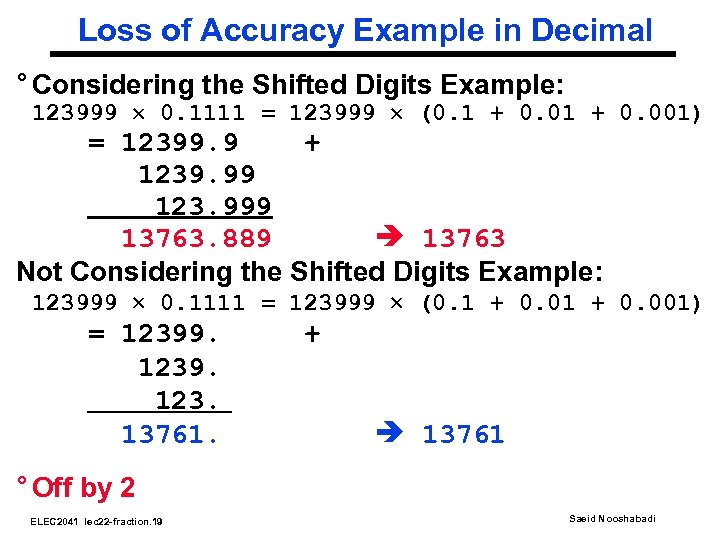Loss of Accuracy Example in Decimal ° Considering the Shifted Digits Example: 123999 0. 1111 = 123999 (0. 1 + 0. 001) = 12399. 9 + 1239. 99 123. 999 13763. 889 13763 Not Considering the Shifted Digits Example: 123999 0. 1111 = 123999 (0. 1 + 0. 001) = 12399. 123. 13761. + 13761 ° Off by 2 ELEC 2041 lec 22 -fraction. 19 Saeid Nooshabadi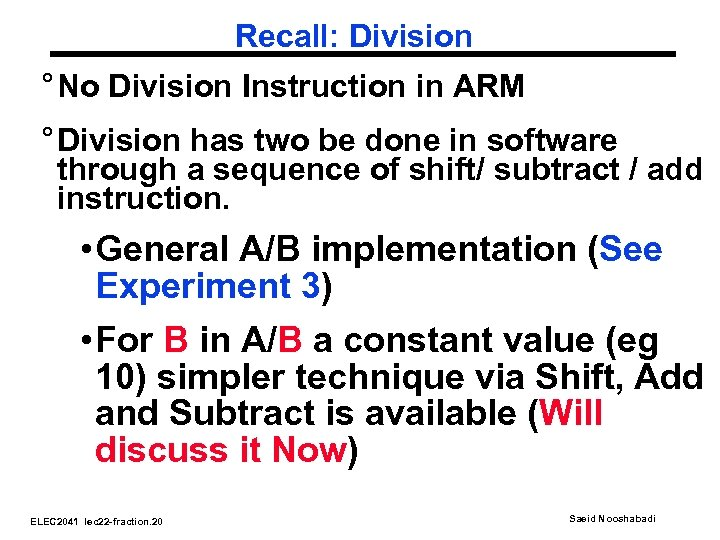Recall: Division ° No Division Instruction in ARM ° Division has two be done in software through a sequence of shift/ subtract / add instruction. • General A/B implementation (See Experiment 3) • For B in A/B a constant value (eg 10) simpler technique via Shift, Add and Subtract is available (Will discuss it Now) ELEC 2041 lec 22 -fraction. 20 Saeid Nooshabadi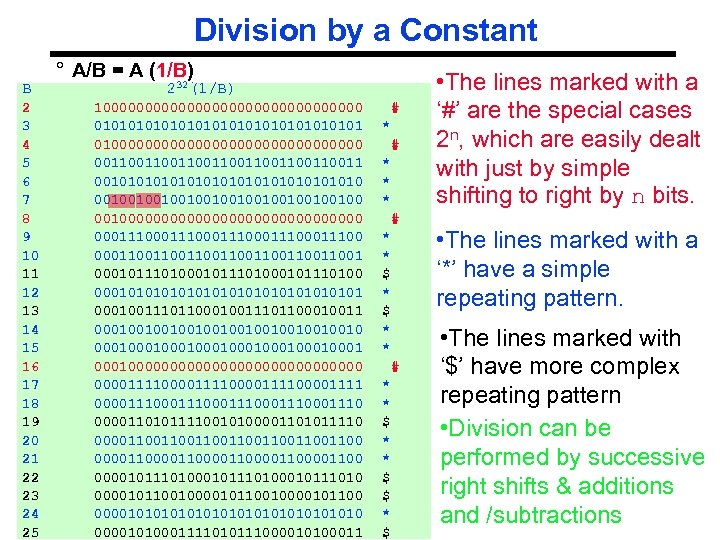Division by a Constant B ° A/B = A (1/B) 2 3 4 5 6 7 8 9 10 11 12 13 14 15 16 17 18 19 20 21 22 23 24 ELEC 2041 25 232(1/B) 10000000000000000 0101010101010101 01000000000000000 0011001100110011 001010101010101010 001001001001001000000000000000 00011100011100011100 00011001100110011001 0001011101000101110100 000101010101010101 00010011101100010011 00010010010010010 000100010001000100000000000000111100001111 00001110001110001110 00001101011110010100001101011110 00001100110011001100 00001100001100001100 0000101110100010111010 000010110010000101100 000010101010101010 lec 22 -fraction. 21 00001010001111010111000010100011 # * * * # * * \$ \$ * \$ • The lines marked with a ‘#’ are the special cases 2 n, which are easily dealt with just by simple shifting to right by n bits. • The lines marked with a ‘*’ have a simple repeating pattern. • The lines marked with ‘\$’ have more complex repeating pattern • Division can be performed by successive right shifts & additions Saeid Nooshabadi and /subtractions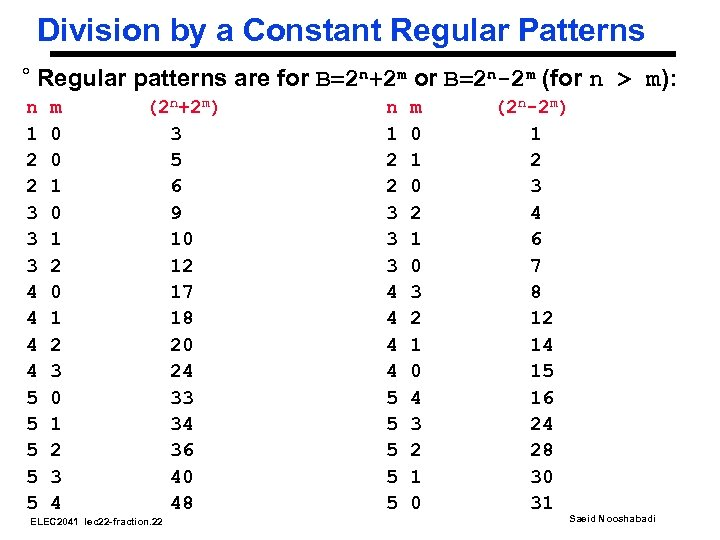Division by a Constant Regular Patterns ° Regular patterns are for B=2 n+2 m or B=2 n-2 m (for n > m): n 1 2 2 3 3 3 4 4 5 5 5 m 0 0 1 2 3 4 (2 n+2 m) 3 5 6 9 10 12 17 18 20 24 33 34 36 40 48 ELEC 2041 lec 22 -fraction. 22 n 1 2 2 3 3 3 4 4 5 5 5 m 0 1 0 2 1 0 3 2 1 0 4 3 2 1 0 (2 n-2 m) 1 2 3 4 6 7 8 12 14 15 16 24 28 30 31 Saeid Nooshabadi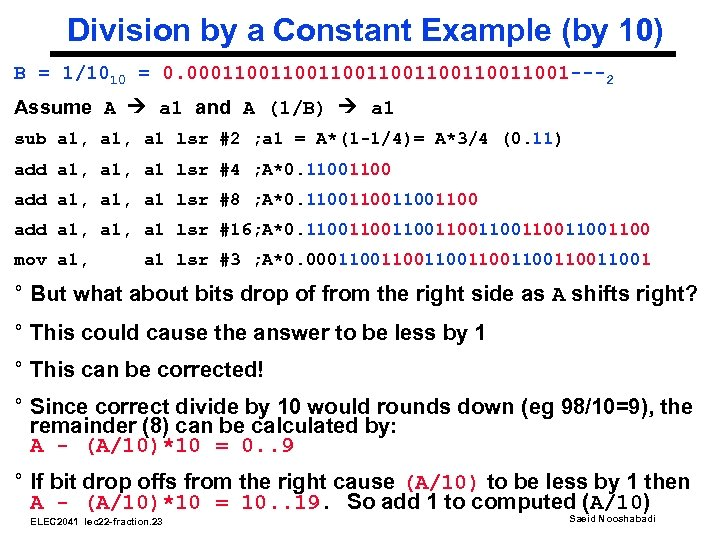Division by a Constant Example (by 10) B = 1/1010 = 0. 00011001100110011001 ---2 Assume A a 1 and A (1/B) a 1 sub a 1, a 1 lsr #2 ; a 1 = A*(1 -1/4)= A*3/4 (0. 11) add a 1, a 1 lsr #4 ; A*0. 1100 add a 1, a 1 lsr #8 ; A*0. 11001100 add a 1, a 1 lsr #16; A*0. 1100110011001100 mov a 1, a 1 lsr #3 ; A*0. 00011001100110011001 ° But what about bits drop of from the right side as A shifts right? ° This could cause the answer to be less by 1 ° This can be corrected! ° Since correct divide by 10 would rounds down (eg 98/10=9), the remainder (8) can be calculated by: A - (A/10)*10 = 0. . 9 ° If bit drop offs from the right cause (A/10) to be less by 1 then A - (A/10)*10 = 10. . 19. So add 1 to computed (A/10) ELEC 2041 lec 22 -fraction. 23 Saeid Nooshabadi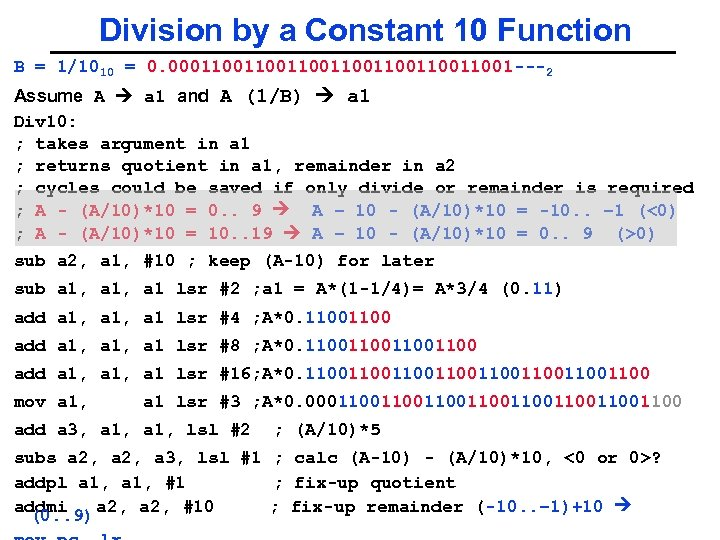Division by a Constant 10 Function B = 1/1010 = 0. 00011001100110011001 ---2 Assume A a 1 and A (1/B) a 1 Div 10: ; takes argument in a 1 ; returns quotient in a 1, remainder in a 2 ; cycles could be saved if only divide or remainder is required ; A - (A/10)*10 = 0. . 9 A – 10 - (A/10)*10 = -10. . – 1 (<0) ; A - (A/10)*10 = 10. . 19 A – 10 - (A/10)*10 = 0. . 9 (>0) sub a 2, a 1, #10 ; keep (A-10) for later sub a 1, a 1 lsr #2 ; a 1 = A*(1 -1/4)= A*3/4 (0. 11) add a 1, a 1 lsr #4 ; A*0. 1100 add a 1, a 1 lsr #8 ; A*0. 11001100 add a 1, a 1 lsr #16; A*0. 1100110011001100 mov a 1, a 1 lsr #3 ; A*0. 0001100110011001100 add a 3, a 1, lsl #2 ; (A/10)*5 subs a 2, a 3, lsl #1 ; calc (A-10) - (A/10)*10, <0 or 0>? addpl a 1, #1 ; fix-up quotient addmi a 2, #10 ; fix-up remainder (-10. . – 1)+10 (0. . 9) Saeid Nooshabadi ELEC 2041 lec 22 -fraction. 24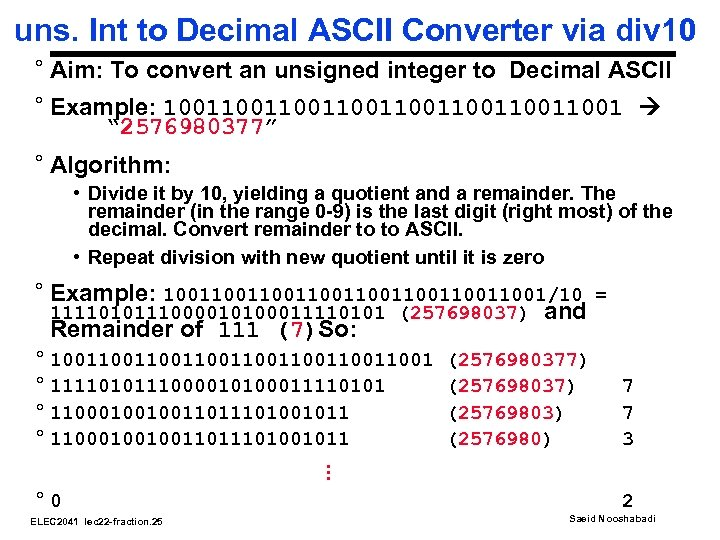uns. Int to Decimal ASCII Converter via div 10 ° Aim: To convert an unsigned integer to Decimal ASCII ° Example: 1001100110011001 “ 2576980377” ° Algorithm: • Divide it by 10, yielding a quotient and a remainder. The remainder (in the range 0 -9) is the last digit (right most) of the decimal. Convert remainder to to ASCII. • Repeat division with new quotient until it is zero ° Example: 1001100110011001/10 = 1111010111000010100011110101 (257698037) and Remainder of 111 (7)So: ° 1001100110011001 ° 1111010111000010100011110101 ° 1100010010011011101001011. . . ° 0 ELEC 2041 lec 22 -fraction. 25 (2576980377) (25769803) (2576980) 7 7 3 2 Saeid Nooshabadi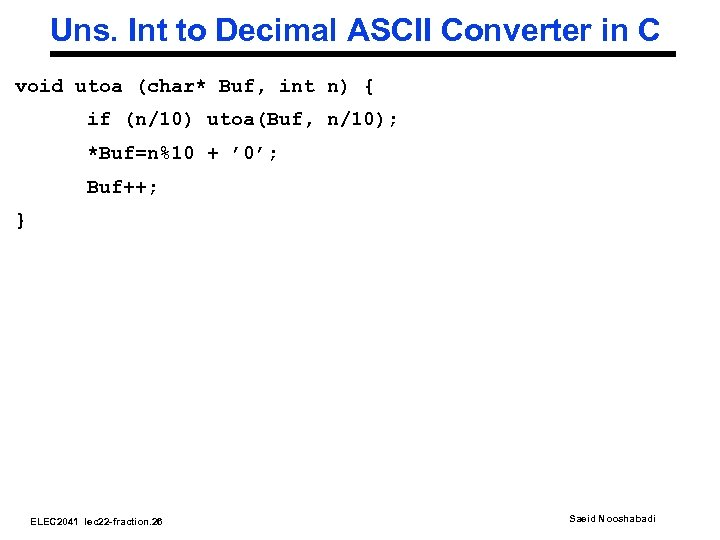Uns. Int to Decimal ASCII Converter in C void utoa (char* Buf, int n) { if (n/10) utoa(Buf, n/10); *Buf=n%10 + ’ 0’; Buf++; } ELEC 2041 lec 22 -fraction. 26 Saeid Nooshabadi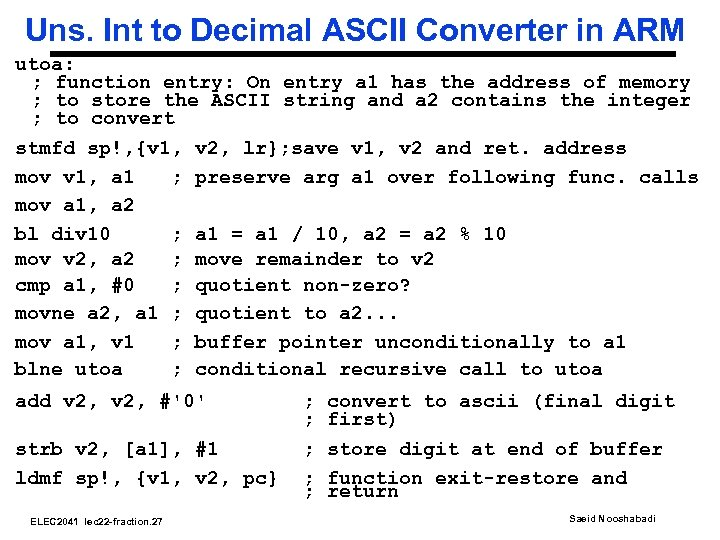Uns. Int to Decimal ASCII Converter in ARM utoa: ; function entry: On entry a 1 has the address of memory ; to store the ASCII string and a 2 contains the integer ; to convert stmfd sp!, {v 1, mov v 1, a 1 ; mov a 1, a 2 bl div 10 ; mov v 2, a 2 ; cmp a 1, #0 ; movne a 2, a 1 ; mov a 1, v 1 ; blne utoa ; v 2, lr}; save v 1, v 2 and ret. address preserve arg a 1 over following func. calls a 1 = a 1 / 10, a 2 = a 2 % 10 move remainder to v 2 quotient non-zero? quotient to a 2. . . buffer pointer unconditionally to a 1 conditional recursive call to utoa add v 2, #'0' ; convert to ascii (final digit ; first) strb v 2, [a 1], #1 ldmf sp!, {v 1, v 2, pc} ; store digit at end of buffer ; function exit-restore and ; return ELEC 2041 lec 22 -fraction. 27 Saeid Nooshabadi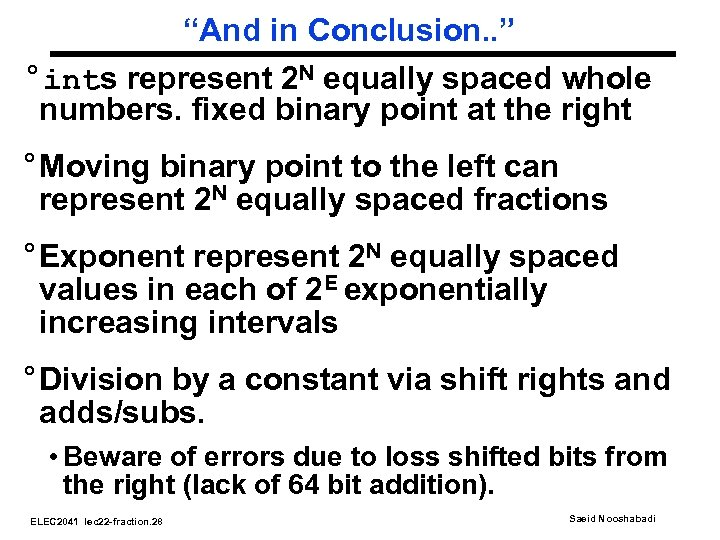“And in Conclusion. . ” °ints represent 2 N equally spaced whole numbers. fixed binary point at the right ° Moving binary point to the left can represent 2 N equally spaced fractions ° Exponent represent 2 N equally spaced values in each of 2 E exponentially increasing intervals ° Division by a constant via shift rights and adds/subs. • Beware of errors due to loss shifted bits from the right (lack of 64 bit addition). ELEC 2041 lec 22 -fraction. 28 Saeid Nooshabadi